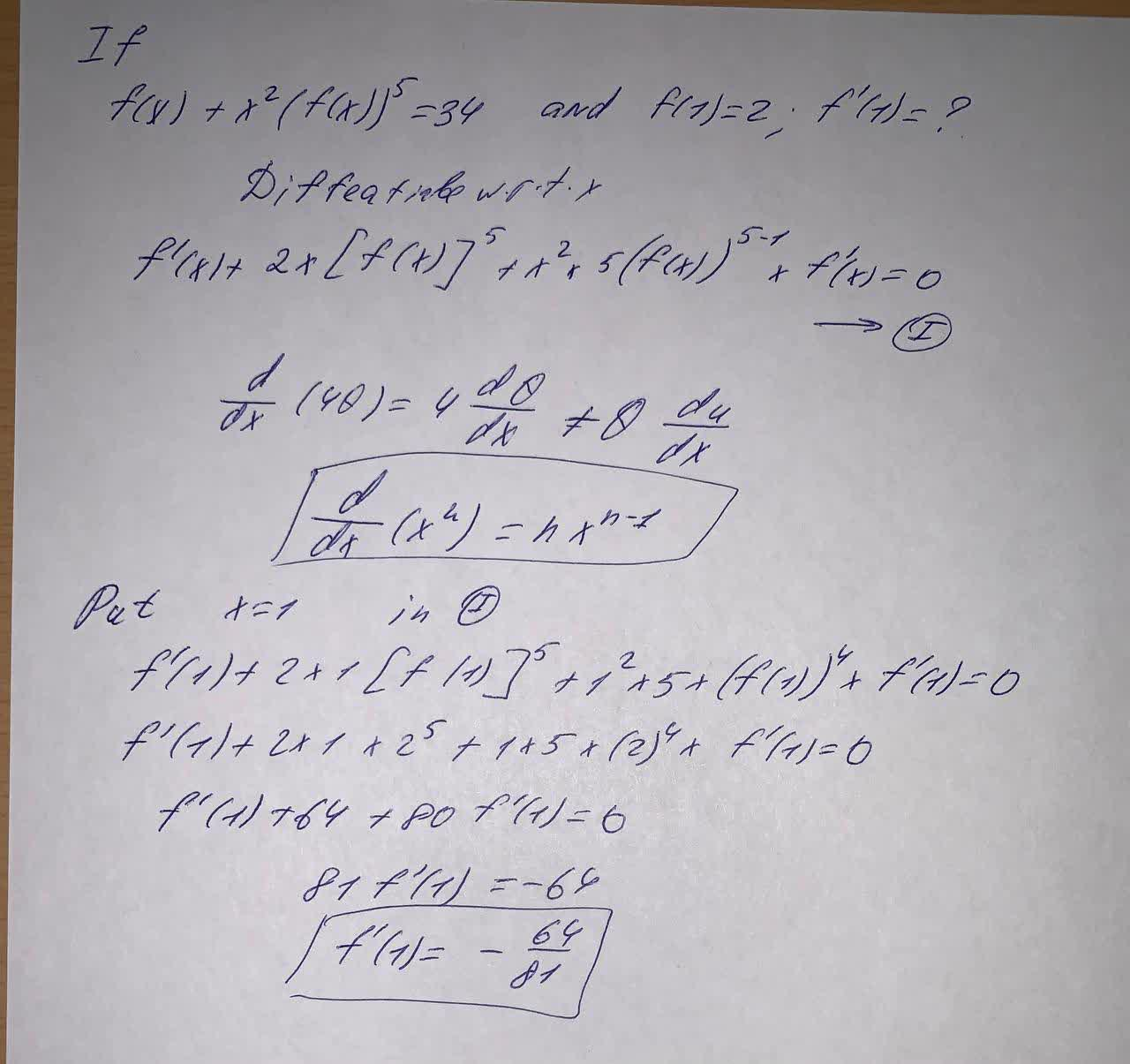Question# If f(x) + x^2[f(x)]^5 = 34 and f(1) = 2, find f '(1).

First order differential equations
ANSWEREDIf $$f(x) + x^2[f(x)]^5 = 34$$ and $$f(1) = 2,$$ find $$f '(1).$$